# Electrical Supervisor - Question and Answer Key

Name of Post: Electrical Supervisor

Department: Apex Societies & MATSYAFED

Cat. Number: 223/2020 & 224/2020

Date of Test: 01.01.2022

Question Code: 001/2022

1. The reciprocal of resistance is
A) Susceptance
B) Conductance
C) Impedance
D) Reactance

2. Two waves of the same frequency have opposite phase when the phase angle between them is
A) 180°
B) 360°
C) 90°
D) 0°

3. The best place to install a capacitor is
A) Very near to inductive load
B) Across the terminals of the inductive load
C) Far away from the inductive load
D) Any where

4. The time constant of a series R-C circuit is given by
A) R/C
B) RC2
C) RC
D) R2C

5. The material  has a negative temperature coefficient of resistance is
A) Aluminium
B) Copper
C) Brass
D) Carbon

6. A dependent source
A) May be a current source or voltage source
B) Is always a voltage source
C) |s always a current source
D) Neither a current source nor a voltage source

7. Capacity of a dry cell is
A) Not affected by the type of discharge
B) More when it supplies current continuously
C) More when it supplies current intermittently
D) None of the above

8. In a lead acid cell, on discharging, both the plates change and become

9. High current of the order of 100 A can be measured by an ammeter of 0 - 1 A rating by using
A) Shunt
B) Capacitor
C) R-C network
D) Current transformer

10. Electrical devices and appliances are not connected in series because
A) More power losses occur in series
B) Series circuit is complex to design
C) Devices have different current rating
D) All of these

11. The force of attraction or repulsion between two magnetic poles is inversely proportional to the square of the distance between them. This is known as
A) Newton's first law
B) Faraday’s first law of electromagnetic induction
C) Coulomb's first law
D) Coulomb's second law

12. Who discovered the relationship between magnetism and electricity that serves as the foundation for the theory of electromagnetism ?
A) Luigi Galvani
B) Hans Christian Oersted
C) Andre Ampere
D) Charles Coulomb

13. A permanent magnet will not attract
A) Copper
B) Steel
C) Nickel
D) Aluminium

14. The unit of magnetomotive force is
A) Weber/metre
B) Ampere turns/metre
C) Ampere turns
D) Weber .

15. Which of the following is a vector quantity ?
A) Flux density
B) Magnetic field intensity
C) Magnetic potential
D) Relative permeability

16. The polarity of induced voltage while a field is collapsing is
A) Independent of the force creating the field
B) Opposite to the force creating the field
C) Identical to the force creating the field
D) Present only if the force is stationary

17. Comparing superparamagnetic and ferromagnetic materials, which statement is false ?
A) Ferromagnetism is usually more powerful than superparamagnetism
B) Ferromagnetism persists when the magnetizing field is removed
C) Superparamagnetism persists once the external field is removed
D) Superparamagnetism can be thought of as a single-domain particle

18. Lower the self inductance of a coil
A) More will be the weber turns
B) More will be the emf induced
C) Lesser the flux produced by it
D) Smaller the delay in establishing steady current through it

19. It is difficult to magnetise steel because of its
A) High density
B) High retentivity
C) Low permeability
D) High permeability

20. The value of dielectric constant for vacuum is taken as
A)1
B) 0
C) 4
D) 10

21. To reduce the error in voltmeter and ammeter due to friction torque
A) The torque weight ratio must be small
B) The weight of moving system must be made as large as possible
C) The torque weight ratio must be large
D) The torque of moving system must be small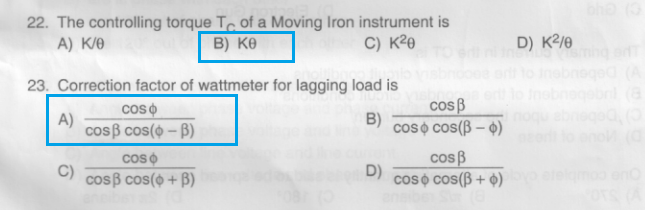24. When 3 phase power is measured by 2 wattmeter method one of the wattmeter will read zero power
A) If the load power factor is unity
B) If the load power factor is zero
C) If the load power factor is less than 0.5
D) If the load power factor is 0.5

25. Light load compensation of integrated type energy meter is done by
B) Breaking magnet
D) Lag plate

26. Single phase electrodynamometer Power factor meter consists of
A) Two identical Pressure coils and one current coil which is split up into two parts
B) One pressure coil and two identical current coils
C) One pressure coil and one current coil
D) None of these

27. Ferro dynamic type frequency meter is a
A) Mechanical resonance type frequency meter
B) Electrical resonance type frequency meter
C) Vibrating type frequency meter
D) Western frequency meter

28. The bridge circuit which measures self inductance in terms of standard capacitor is
A) Anderson's bridge
B) Owen's bridge
C) De Sauty's bridge
D) Maxwell bridge

29. In a CRO intensity of electron beam is controlled by
A) Focusing anode
B) Accelerating anode
C) Grid
D) Electron Gun

30. The primary current in the CT is
A) Dependent of the secondary circuit conditions
B)Independent of the secondary circuit conditions
C) Depends upon the secondary burden
D) None of these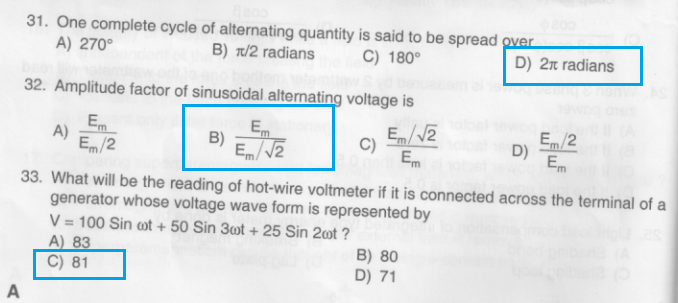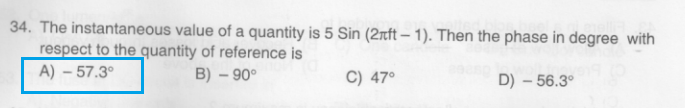35. When pure inductive coil is connected to an AC supply then the average demand of power from power supply is
A) Zero
B) Maximum
C) Minimum
D) None of these

37. The current in a pure capacitor leads its voltage by
A) Half cycle
B) Full cycle
C) Quarter cycle
D) None of these

38. When three phase symmetrical balanced load is connected to 3 phase 4 wire star system, the neutral wire will be carrying three currents which are exactly equal in magnitude but
A) are 240° out of phase with each other
B) are in phase with each other
C) are 90° out of phase with each other
D) are 120° out of phase with each other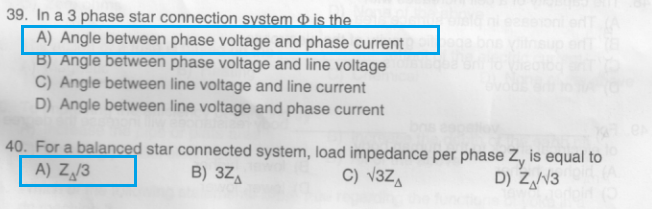41. The efficiency of a solar cell is between
A) 5% to 10%
B) 15% to 20%
C) 25% to 40%
D) 50% to 60%

42. The purpose of earthing the electrical equipment is
A) To bring it to zero potential
B) To avoid shock to the operator
C) Both A) and B)
D) None of these

43. Fillers in a lead acid battery are provided to
A) Allow flow of gases
B) Recover acid losses through vapour
C) Prevent flow of gases
D) None of the above

44. In which solar energy collector the efficiency is maximum ?
A) Evacuated tube
B) Line focusing
C) Flat plate
D) Paraboloid dish

45.The primary purpose of a grounding system is to provide a ___________ path for currents to the earth.
A) High resistance
B) Low impedance
C) High impedance
D) None of the above

46. Two 12 Volt 60 Ah batteries are connected in parallel, we get __________ output.
A) 12 Volt 120 Ah
B) 24 Volt 60 Ah
C) 24 Volt 120 Ah
D) 12 Volt 30 Ah

47. Hydrometer is used to measure the ____________ in a lead acid cell.
A) Current rating
B) Specific gravity of electrolyte
C) Power rating
D) Efficiency

48. The capacity of a cell increases with
A) The increase in plate surface area
B) The quantity and specific gravity of the electrolyte
C) The porosity of the separators
D) All of the above

49. For ____________  voltages and _______________ body resistances will increase the degree of electrical shock to the human body.
A) higher, higher
B) lower, higher
C) higher, lower
D) lower, lower

50. Which of the following device will not operate at fault ?
A) lsolator
B) Circuit breaker
C) Fuse
D) MCB

51. Melting point of Tungsten is
A) 2400°C
B) 2700°C
C) 3000°C
D) 3400°C

52. One lumen/m2=
A) One lux
B) Coulomb
C) One candela
D) One foot candle

53. The fuse in DC circuit is inserted in
A) Negative line only
B) Positive line only
C) Both negative and positive lines
D) Either negative or Positive line

54. The unit of solid angle is
A) Lumen
B) Lux
C) Degree

55. Carbon arc lamps are commonly used in
A) Street lighting
B) Cinema hall lighting
C) Cinema projector
D) Domestic lighting

56. The HRC fuses are standardized for rated current up to _______ Ampere,
A) 500
B) 1000
C) 1250
D) 2500

57. Current rating of 1.5 mm? single core copper wire is ________ Amps.
A)5
B) 10
C) 15
D) 20

58. The earth resistance of a domestic wiring is
A) Less than 5 ohms
B) More than 5 ohms
C) Zero ohms
D) None of the above

59. The action of a fuse is based on the __________ effect of the electric current.
A) Magnetic
B) Heating
C) Chemical
D) None of the above

60. To improving the efficiency of earthing by
A) Increase the pipe or plate area
B) Increase the depth of the earth pit
C) Installing parallel electrodes
D) All of the above

61.Which of the following statements is/are true regarding the functions of yoke in a dc machine ?
i. It provides path for the pole flux.
ii. It provides mechanical Support to the machine.
iii. It helps to convert the AG EMF to DC.
iv. It helps in reducing the armature reaction effect.
A) Only i and ii
B) Only i, ii and iii
C) Only i and iv
D) Only ii, iii and iv

62. The number of parallel paths in a 4 pole wave wound de machine is
A) 4
B) 2
C) 8
D) 1

63. Inorder to obtain maximum generated EMF in the armature of a de generator, what should be the shape of field flux waveform ?
A) Flat topped waveform
B) Sinusoidal waveform
C) Triangular
D) Ramp shaped

64. Match the following starters and the motors in which they are employed ?
I                                                    II
1. Three pointer starter       i. DC shunt motor with field control
2. Four point starter           ii. DC shunt motor without field control
3. Two point starter           iii. DC series motor
A) 1-ii, 2-i, 3-iii
B) 1-i, 2-ii, 3-iii
C) 1-iii, 2-ii, 3-i
D) 1-i, 2-iii, 3-ii

65. Incase of compound de machines, the reason/reasons for keeping the series field wound over the shunt field coil is/are
A) Convenience in construction
B) For better cooling
C) To improve commutation
D) Both (A) and (B)

66. The main reason for improper commutation in case of de machine is
A) Inductance of armature winding
B) Use of interpoles
C) Use of compensating winding
D) Field flux

67. A long shunt de compound generator delivers 2.2 kW at 220 V. If armature resistan is 0.1 ohms, series field winding resistance is 0.2 ohms and shunt field resistance is 110 ohms, calculate the value of armature current in amperes.
A) 10A
B) 8A
C) 12A
D) 6A

68. Which of the following statements is/are true regarding mechanical characteristic of a de shunt motor ?
i. Itis the graph between torque and speed of motor.
ii. Itis the graph between armature current and armature torque.
iii. The shape of the characteristic is linear.
iv. The shape of the characteristics is hyperbolic.
A) Only i and iv
B) Only ii and iii
C) Only i and iii
D) Only ii and iv

69. During the no load test on a 200 V, DC shunt motor having armature resistance of 0.1 Ω and field resistance of 200 Ω, the armature current is recorded to be 10 A. The constant loss of the motor is
A) 2000 W
B) 1990 W
C) 2200 W
D) 2199 W

70. ADC shunt motor is running at 1500 rpm. An additional resistance is introduced in the field Circuit of the motor without any change in applied voltage. The speed of the motor is
A) Will remain the same
B) Will be more than 1500 rpm
C) Will be less than 1500 rpm
D) Will reduce to zero

71. Ifthe input supply frequency of a transformer is doubled, keeping the input voltage constant which of the following statements are true ?
i. Core loss gets doubled.
ii. Eddy current loss gets doubled.
iii. Hysteresis loss reduces.
A) Only i and ii
B) Only iii
C) Only i
D) Only ii

72. The purpose of using laminations in a transformer is to reduce
A) Eddy current loss
B) Hysteresis loss
C) Copper loss
D) Friction and windage loss

73. The rotor EMF frequency of a 3 phase induction motor is found to be 2 Hz and the input Supply frequency to the motor is 50 Hz. Calculate the percentage slip of the motor.
A) 6%
B) 8%
C) 4%
D) 5%

74. Which of the following techniques can be used for speed control of three phase squirrel cage induction motor ?
i. Rotor resistance control.
ii. V/f control.
iii. Pole changing method.
A) Only i and ii
B) Only i and iii
C) Only ii
D) Only ii and iii

75. Which of the following statements is/are true regarding star delta starter of three phase induction motor ?
i. At the time of starting the windings are connected in star and after attaining the steady state speed the windings are connected in delta.
ii. At the time of starting the windings are connected in delta and after attaining the steady state speed the windings are connected in star.
iii. It reduces the starting torque to one third of that produced by direct switching in delta.
iv. It increases the starting torque to three times of that produced by direct switching in delta.
A) Only i and iii
B) Only ii and iii
C) Only i and iv
D) Only ii and iv

76. The number of slip rings in a star connected three phase alternator with the field provided on rotor is
A) 3
B) 4
C)2
D) 5

77. The armature reaction effect in a three phase alternator operating in isolated condition depends on which of the following ?
i. Power factor of the load.
ii. Speed of the machine.
A) Only i and iii
B) Only ii and iii
C) Only i
D) Only iii

78. Which of the following statement/statements are true regarding damper winding in a three phase synchronous motor ?
i, Damper winding helps to start the motor.
ii, Current is present in damper winding when the motor is running at synchronous speed.
iii. Current is zero in damper winding when the motor is running at synchronous speed.
A) Only iii
B) Only i and ii
C) Only i
D) Only i and iii

79. The direction of rotation of a single phase induction motor can be reversed by
A) Reversing the leads of the supply
B) Reversing the leads of main winding
C) Reversing the leads of starting winding
D) Either B or C

80. Which of the following can be employed for speed control of universal motors ?
i. Resistance connected in series to armature.
i. Tappings provided on field winding.
ili. Using centrifugal device.
A) Only i and iii
B) Only ii and iii
C) Only ii
D) i, ii and iii

81. The power delivered to the load is maximum when the SCR firing angle is
A) 90°
B) 180°
C) Zero
D) Between 90° and 180°

82. A UJT has Reg = 15 K ohms, RB, = 5 K ohms. Its intrinsic stand off ratio is
A) 0.67
B) 0.5
C) 0.8
D) 0.33

83. The operation of JFET involves
A) Recombination
B) Flow of minority carriers
C) Negative resistance
D) Flow of majority carriers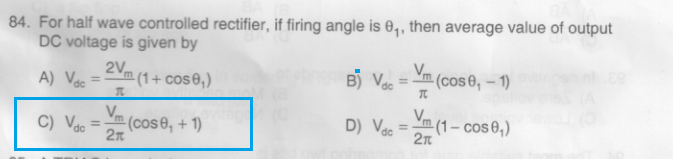85. A TRIAC is equivalent to two SCRs
A) In series
B) In inverse parallel
C) In parallel
D) In inverse series

86. Which is an example of DIAC ?
A) NTE 6408
B) OptoNCD ILR 1030
C) EddyNCD 3301
D) BT 136

87. A latched up IGBT can be tumed off by
A) UJT triggering circuit
B) Forced commutation of voltage
C) Snubber circuit
D) Forced commutation of current

88. A single phase half bridge inverter has input supply voltage of 100 volts. For a load resistance of 5 ohms, the output power is equal to (square wave output)
A) 2000 watts
B) 500 watts
C) 1000 watts
D) 750 watts

89. The internal resistance of an ideal voltage source is
A) High
B) Moderate
C) Zero
D) Very high

90. _________ is used in the rotating type UPS system to supply the mains.
A) Battery bank
B) DC motor
C) Self excited DC generator
D) Alternator

91. The decimal number — 19 is expressed in 2’s complement form as
A) 10010011
B) 11101101
C) 11011100
D) 00110011

93. In negative logic, logic state 1 corresponds to
A) Zero voltage
B) More negative voltage
C) Lower voltage level
D) Negative voltage

94. The most suitable gate for comparing two bits is
A) NAND
B) XOR
C) AND
D) OR

95. A feature that distinguishes the JK flip flop from the SR flip flop is the
A) Type af clock
B) Clear input
G) Present input
D) Toggle condition

96. In a 4 bits weighted resistor D/A converter, the resistor value corresponding to LSB is 64 K Ohms. The resistor value corresponding to MSB will be
A) 4K Ohms
B) 64 K Ohms
C) 8K Ohms
D) 16 K Ohms

97. The disadvantage of counter type A/D converter as compared to comparator type A/D converter is that
A) Longer conversion time is required
B) The resolution is low
C) Its stability is low
D) The circuit is more complex

98. A divide by 54 counter can be realized by
A) 9 numbers of mod 9 counters
B) 6 numbers of mod 9 counters
C) 9 numbers of mod 6 counter
D) one mod 9 counter followed by one mod 6 counter

99. A stage in a shift register consists of
A) a latch
B) a byte of storage
C) a flip flop
D) four bits of storage

100. The purpose of using flux in soldering is to
A) Fill up gaps left in a bad joint
B) Prevent oxides forming
C) Wash away surplus solder
D) Increase fluidity of solder metal